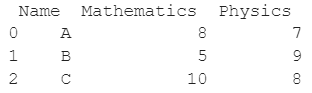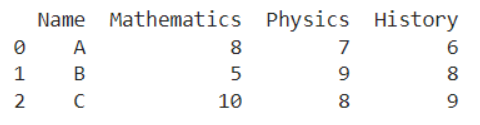# How To Insert A Column At A Specific Column Index In Pandas?

A DataFrame is the primary data structure of the Pandas library and is commonly used for storing and working with tabular data.

A common operation that could be performed on such data is to insert a column at a specific index in order to add more information to it.

To start working with Pandas, we first need to import it by using the below code:

``import pandas as pd``

## Running Example

Let us understand this operation with the help of an example. Consider the following DataFrame containing 3 students with names A, B, and C and their corresponding marks (out of 10) for two subjects, Mathematics and Physics.Code snippet for generating the above DataFrame:

``````import pandas as pd
# Dictionary for our data
data = {'Name' : ['A', 'B', 'C'], 'Mathematics' : [8, 5, 10], 'Physics' : [7, 9, 8]}
# DataFrame for the dictionary
df = pd.DataFrame(data)
# Printing the DataFrame
print(df)``````

Here, data is a dictionary, we created to initialize the DataFrame. For this, we use the DataFrame() function of the Pandas library which takes the dictionary as an argument and returns the required DataFrame.

Now, let’s say, we need to add the marks of another subject, History, to this DataFrame as shown below at index 3. The resulting DataFrame would look like this:Let us look at different ways of inserting a column at a specific index in a given DataFrame:

## Inserting Column in Dataframe using a list in Pandas

This method is pretty straightforward and is the most commonly used one. The syntax can be seen below, with History being the new column’s label and [6,8,9] being a list denoting row-wise values for that column.

The resulting new column is added as the last one in the DataFrame.

This method is used for the in-place addition of a column in the DataFrame. Note that this method only works if the desired index is at the last place. The order of columns can later be changed if required.

``````import pandas as pd
# Dictionary for our data
data = {'Name' : ['A', 'B', 'C'], 'Mathematics' : [8, 5, 10], 'Physics' : [7, 9, 8]}
# DataFrame for the dictionary
df = pd.DataFrame(data)
# Adding a new column named History
df['History'] = [6, 8, 9]
# Printing the new DataFrame
print(df)``````

Output:## Insert Column in Dataframe using insert() function in Pandas

In this method, we use the insert() function to add a new column to an existing DataFrame.

This function can be used to add the column at any position, not necessarily at the end of the DataFrame.

This method is used for the in-place addition of a column in the DataFrame. The new column’s index, label, and data can be specified as function arguments as follows (3, ‘History’ and [6,8,9] respectively here):

``````import pandas as pd
# Dictionary for our data
data = {'Name' : ['A', 'B', 'C'], 'Mathematics' : [8, 5, 10], 'Physics' : [7, 9, 8]}
# DataFrame for the dictionary
df = pd.DataFrame(data)
# Adding a new column named History
df.insert(3, 'History', [6,8,9])
# Printing the new DataFrame
print(df)``````

Output:If the label of the column to be added matches that of another column already present in the DataFrame, we receive an error. For such additions, it is better to use the assign() function instead. Note that this method only works if the desired index is at the last place.

## Insert Column in Dataframe using assign() function in Pandas

In this method, we use the assign() function to add a new column to an existing DataFrame. This function returns the updated DataFrame. The syntax of the argument is as follows:

``<name_of_col_to_be_added> = <value>``

Existing columns that are reassigned will be overwritten. Note that this method only works if the desired index is at the last place. The order of columns can later be changed if required.

``````import pandas as pd
# Dictionary for our data
data = {'Name' : ['A', 'B', 'C'], 'Mathematics' : [8, 5, 10], 'Physics' : [7, 9, 8]}
# DataFrame for the dictionary
df = pd.DataFrame(data)
# Adding a new column named History
df = df.assign(History = [6,8,9])
# Printing the new DataFrame
print(df)``````

Output:## Insert Column in Dataframe using .loc property in Pandas

In this python code, we shall use the .loc property of DataFrames to add a new column.

Through :(colon), we specify that we need to add values for all rows and in the second input we specify the required column label.

This method is used for the in-place addition of a column. Note that this method only works if the desired index is at the last place. The order of columns can later be changed if required.

``````import pandas as pd
# Dictionary for our data
data = {'Name' : ['A', 'B', 'C'], 'Mathematics' : [8, 5, 10], 'Physics' : [7, 9, 8]}
# DataFrame for the dictionary
df = pd.DataFrame(data)
# Adding a new column named History
df.loc[:, 'History'] = [6,8,9]
# Printing the new DataFrame
print(df)``````

Output:## Insert Column in Dataframe using eval() function in Pandas

In this method, we use the eval() function to add a new column to the DataFrame. We specify the argument as a string expression in the following manner:

``<new_col_label> = <new_col_value>``

The values of the new column can be specified using a list. The list [6,8,9] was used as an example for this purpose.

The eval() function returns the updated DataFrame. Note that this method only works if the desired index is at the last place. The order of columns can later be changed if required.

``````import pandas as pd
# Dictionary for our data
data = {'Name' : ['A', 'B', 'C'], 'Mathematics' : [8, 5, 10], 'Physics' : [7, 9, 8]}
# DataFrame for the dictionary
df = pd.DataFrame(data)
# Adding a new column named History
df = df.eval('History = [6,8,9]')
# Printing the new DataFrame
print(df)``````

Output:By default, the addition of a new column is not inplace. For inplace addition you must set the parameter inplace = True as follows:

``````import pandas as pd
# Dictionary for our data
data = {'Name' : ['A', 'B', 'C'], 'Mathematics' : [8, 5, 10], 'Physics' : [7, 9, 8]}
# DataFrame for the dictionary
df = pd.DataFrame(data)
# Adding a new column named History
df.eval('History = [6,8,9]', inplace = True)
# Printing the new DataFrame
print(df)``````

Output:## Conclusion

In this topic, we have learned to insert a column at a specific index in a column of an existing DataFrame, following a running example of test scores of students in different subjects, thus giving us an intuition of how this concept could be applied in real-world situations.

Trending